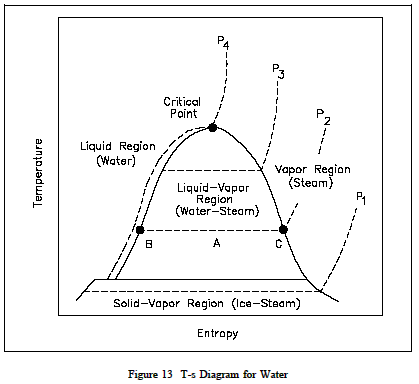Temperature Entropy (T-s) Diagram - Thermodynamics

Temperature Entropy (T-s) Diagram

A T-s diagram is the type of diagram most frequently used to analyze energy transfer system cycles. This is because the work done by or on the system and the heat added to or removed from the system can be visualized on the T-s diagram. By the definition of entropy, the heat transferred to or from a system equals the area under the T-s curve of the process. Figure 13 is the T-s diagram for pure water. A T-s diagram can be constructed for any pure substance. It exhibits the same features as P-u diagrams.

Temperature Entropy (T-s) DiagramIn the liquid-vapor region in Figure 13, water and steam exist together. For example, at point A, water with an entropy (sf) given by point B, exists together with steam with an entropy (sg) given by point C. The quality of the mixture at any point in the liquid-vapor region can be found using the following relationship.Membership Register | LoginHomeEngineering Book StoreEngineering ForumExcel App. DownloadsOnline Books & ManualsEngineering NewsEngineering VideosEngineering CalculatorsEngineering ToolboxGD&T Training Geometric Dimensioning TolerancingDFM DFA TrainingTraining Online EngineeringAdvertising CenterCopyright Notice